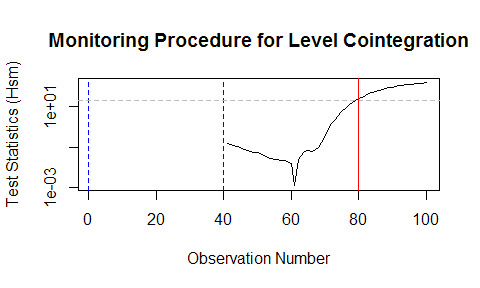# cointmonitoR

## Install cointmonitoR

Since it’s not available on CRAN yet, you have to download and install the package directly from GitHub. Also, the latest version of our cointReg package has to be installed. It provides functions for parameter estimation and inference in cointegrating regressions and the cointmonitoR package depends on it. See its CRAN page for further information.

install.packages("cointReg")
devtools::install_github("aschersleben/cointmonitoR", build_vignettes = TRUE)
library("cointmonitoR")

## Basic examples

### Monitoring Stationarity

Generate a time series to monitor. Since it’s the easiest way to generate a stationary time series, we get x1 as a vector of random number from a standard normal distribution.

set.seed(1909)
x1 <- rnorm(200)

The use of the monitoring procedure is really simple, you just have to choose the length of the calibration period. Here we take m as the first 25 % of the total length of x1 (i.e. the first 50 observations).

test1 <- monitorStationarity(x1, m = 0.25)

Now you can print the results:

print(test1)
##
## ### Monitoring Procedure for Level Stationarity ###
##
## Model:       x1
##
## Parameters:  m = 0.25 (last observation used for calibration: 50)
##      Kernel = "ba"  //  Bandwidth = 1.104631 (Andrews)
##
## Results: Test Statistic (Hsm) = 0.4437515
##      p-Value >= 0.1 (not significant to 0.05 level)

…and also plot it. Tell the plot method what you want to see – the "test" statistics (default) or the "values" / "residuals" of the tested time series or "both" (which will generate two plots at once).

plot(test1, what = "test")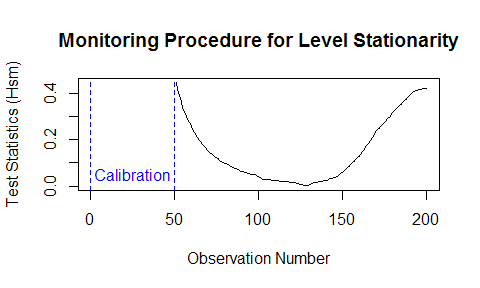Let’s also have a look at an example, where (obviously) a structural break occurs and the stationarity ends after 100 observations. You may want exactly the first 93 observations to be part of the calibration period – which is no problem, since you can just specify it via the m argument in both ways (fraction of length and number of observations).

x2 <- c(x1[1:100], cumsum(x1[101:200]) / 2)
test2 <- monitorStationarity(x2, m = 93)
print(test2)
##
## ### Monitoring Procedure for Level Stationarity ###
##
## Model:       x2
##
## Parameters:  m = 0.465 (last observation used for calibration: 93)
##      Kernel = "ba"  //  Bandwidth = 1.552963 (Andrews)
##
## Results: Test Statistic (Hsm) = 5.026546
##      p-Value <= 0.01 (significant to 0.05 level)
##      Rejection Time = 126

As already mentioned above, you can set the what argument to "both" to get to plots from the model:

oldpar <- par(mfrow = c(2, 1))
plot(test2, what = "both", legend = FALSE)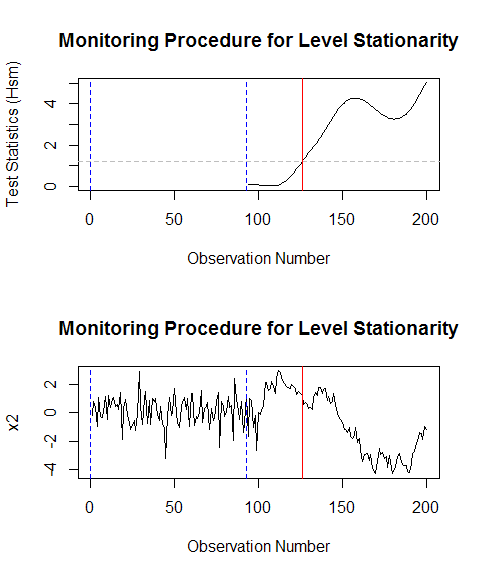par(oldpar)

### Monitoring Cointegration

We generate a cointegration model with three regressors and get the response variable as the sum of them plus an intercept term of 1 and a random error.

set.seed(1909)
x1 <- cumsum(rnorm(100, mean = 0.05, sd = 0.1))
x2 <- cumsum(rnorm(100, sd = 0.1)) + 1
x3 <- cumsum(rnorm(100, sd = 0.2)) + 2
x <- cbind(x1, x2, x3)
y <- 1 + x1 + x2 + x3 + rnorm(100, sd = 0.2)
matplot(1:100, cbind(y, x), type = "l", main = "Cointegration Model",
xlab = "Observation Number", ylab = "")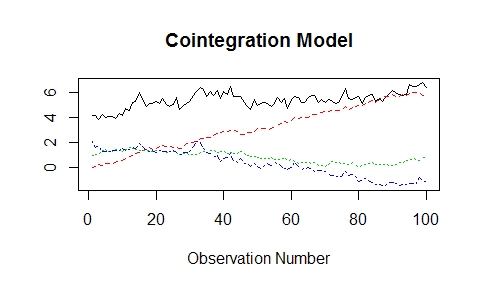Now, you want to monitor the cointegrating relationship and set the calibration period to 40 observations. There are (at least) two additional questions that you have to think about:

1. Which method to use for estimating the model parameters?
This is done with the cointReg package, so you first of all may have a look at its documentation. There are three possible choices: "FM", "D" and "IM".

2. Include a linear trend or only use an intercept?
For this question it may be helpful to see the results of a “full” cointReg model:

m <- 40
cointRegFM(x[1:m, ], y[1:m], deter = cbind(level = 1, trend = 1:m))
##
## ### FM-OLS model ###
##
## Model:       y[1:m] ~ cbind(level = 1, trend = 1:m) + x[1:m, ]
##
## Parameters:  Kernel = "ba"  //  Bandwidth = 2.053036 ("Andrews")
##
## Coefficients:
##        Estimate   Std.Err t value Pr(|t|>0)
## level 1.0602145 0.4445214  2.3851 0.0226240 *
## trend 0.0069304 0.0153027  0.4529 0.6534249
## x1    0.8707882 0.2105639  4.1355 0.0002106 ***
## x2    0.9704294 0.2608974  3.7196 0.0006967 ***
## x3    0.9829914 0.1061508  9.2603 6.087e-11 ***
## ---
## Signif. codes:  0 '***' 0.001 '**' 0.01 '*' 0.05 '.' 0.1 ' ' 1

In the calibration period, the linear trend component is not significantly different from 0, so it should be sufficient to set trend = FALSE.

test3 <- monitorCointegration(x = x, y = y, m = m, model = "FM", trend = FALSE)
print(test3)
##
## ### Monitoring Procedure for Level Cointegration ###
##
## Model:       y ~ x
##
## Parameters:  m = 0.4 (last observation used for calibration: 40)
##      Model = FM-OLS  //  Kernel = "ba"  //  Bandwidth = 2.070899 (Andrews)
##
## Results: Test Statistic (Hsm) = 0.1476303
##      p-Value >= 0.1 (not significant to 0.05 level)

Again, this can be plotted to get a visual understanding of the results:

oldpar <- par(mfrow = c(2, 1))
plot(test3, what = "both", legend = FALSE)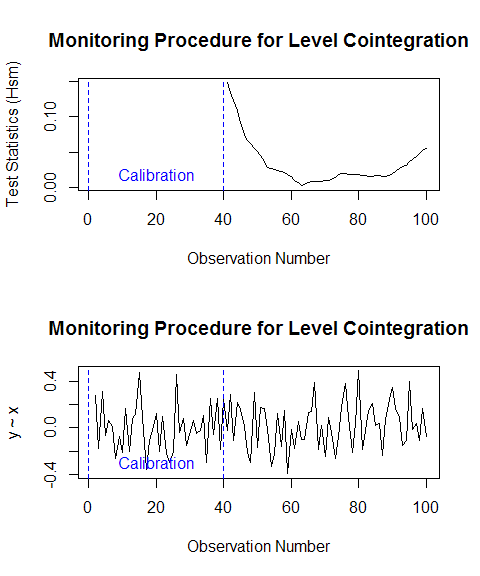par(oldpar)

Obviously, the test statistics are always on a very low level and the residuals in the post-calibration period, determined by using the calibration period FM-OLS model, do not differ from its former behaviour.

Finally, we can investigate a “broken” cointegration model, like the following one. It’s with the same x and y values as above, but with an additional random-walk term beginning with observation no. 61.

y2 <- y + c(rep(0, 60), cumsum(rnorm(40, sd = 0.5)))
matplot(1:100, cbind(y2, x), type = "l", main = "Cointegration Model",
xlab = "Observation Number", ylab = "")
abline(v = 60.5, col = 2)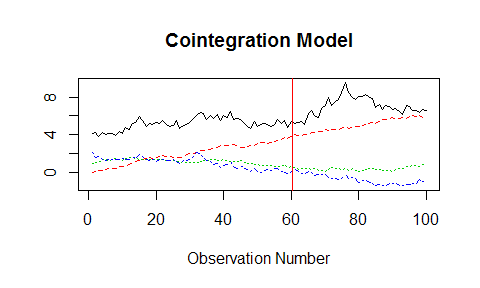Again, we set the argument trend = FALSE and have a look at the monitoring results:

test4 <- monitorCointegration(x = x, y = y2, m = m, model = "FM", trend = FALSE)
print(test4)
##
## ### Monitoring Procedure for Level Cointegration ###
##
## Model:       y2 ~ x
##
## Parameters:  m = 0.4 (last observation used for calibration: 40)
##      Model = FM-OLS  //  Kernel = "ba"  //  Bandwidth = 2.070899 (Andrews)
##
## Results: Test Statistic (Hsm) = 156.1611
##      p-Value <= 0.01 (significant to 0.05 level)
##      Rejection Time = 80

We can see, that the procedure is able to detect this structural break on a significant level (p < 0.01).

The plot shows that the test statistic is increasing and crosses the critical value (grey dashed line) at observation no. 80.

oldpar <- par(mfrow = c(2, 1))
plot(test4, what = "both", legend = FALSE)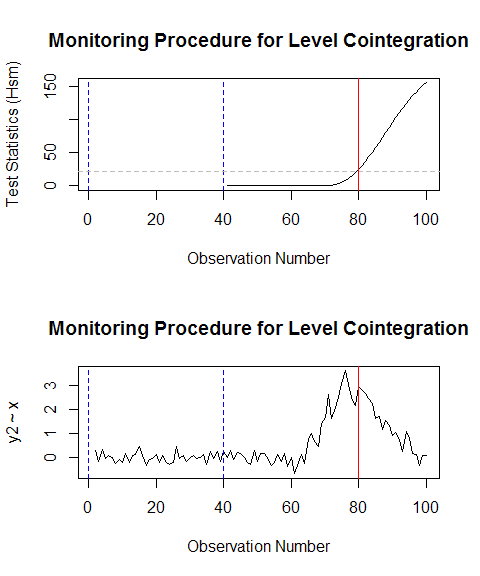par(oldpar)

For a detailed view on the test statistics, you can use the base plot argument log. It reveals that the test statistic is starting to grow some observations after no. 61, which is the first one after the structural break.

plot(test4, what = "test", legend = FALSE, log = "y")